# Gluing lemma for open subsets

## Statement

Let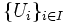$\{U_i\}_{i \in I}$ be a collection of open subsets of a topological space$X$, and$f_i:U_i \to Y$ be continuous maps, such that for$x \in U_i \cap U_j$ we have$f_i(x) = f_j(x)$.

Let$U$ be the union of the$U_i$s. Then there exists a unique map$f:U \to Y$ such that$f|_{U_i} = f_i$.

This is the proof that the presheaf of continuous functions to$Y$, is actually a sheaf.

## Proof

The key facts used in the proof are:

• A map of topological spaces is continuous iff the inverse image of any open set is open
• An open subset of an open subset is open in the whole space
• An arbitrary union of open subsets is open

### Proof details

Given: An open cover$\{ U_i \}_{i \in I}$ of a topological space$X$. Continuous maps$f_i:U_i \to Y$, such that for$x \in U_i \cap U_j$, we have$f_i(x) = f_j(x)$.$U$ is the union of the$U_i$s.

To prove: There exists a unique map$f:U \to Y$ such that$f|_{U_i} = f_i$.

Proof: Note first that the$U_i$s are all open in$X$, hence also in$U$.

1. There exists a unique function$f$ on$U$ such that$f|_{U_i} = f_i$ for all$i$: For any$x \in X$, pick any$i$ such that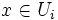$x \in U_i$, and define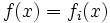$f(x) = f_i(x)$. Such an$i$ exists because$U$ is the union of the$U_i$s. Further, the definition of$f(x)$ is independent of the choice of$i$ because if$x \in U_i \cap U_j$,$f_i(x) = f_j(x)$. Moreover, this is the only possible way to define$f$.
2.$f$ is continuous, i.e., if$V$ is an open subset of$Y$,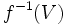$f^{-1}(V)$ is an open subset of$U$: If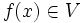$f(x) \in V$, then$f_i(x) \in V$ for some$i$. Thus, we have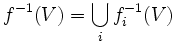$f^{-1}(V) = \bigcup_i f_i^{-1}(V)$. Since$f_i:U_i \to Y$ is continuous,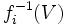$f_i^{-1}(V)$ is open in$U_i$. Since open subsets of open subsets are open, and$U_i$ is open in$U$,$f_i^{-1}(V)$ is open in$U$. Thus, the union$f^{-1}(V)$ of all the$f_i^{-1}(V)$ is also an open subset of$U$.